# Adaptive MPC Control of Nonlinear Chemical Reactor Using Online Model Estimation

This example shows how to use an Adaptive MPC controller to control a nonlinear continuous stirred tank reactor (CSTR) as it transitions from low conversion rate to high conversion rate.

A discrete time ARX model is being identified online by the Recursive Polynomial Model Estimator block at each control interval. The adaptive MPC controller uses it to update internal plant model and achieves nonlinear control successfully.

### About the Continuous Stirred Tank Reactor

A Continuously Stirred Tank Reactor (CSTR) is a common chemical system in the process industry. A schematic of the CSTR system is: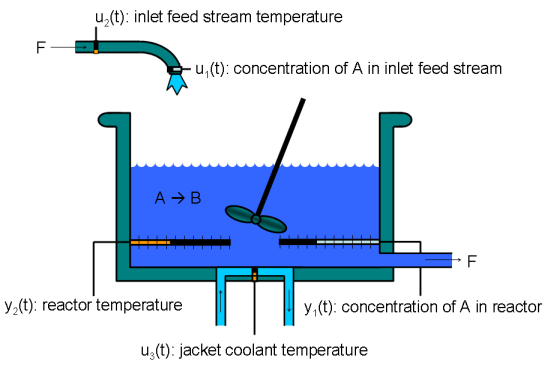This is a jacketed non-adiabatic tank reactor described extensively in Seborg's book, "Process Dynamics and Control", published by Wiley, 2004. The vessel is assumed to be perfectly mixed, and a single first-order exothermic and irreversible reaction, A --> B, takes place. The inlet stream of reagent A is fed to the tank at a constant volumetric rate. The product stream exits continuously at the same volumetric rate and liquid density is constant. Thus the volume of reacting liquid is constant.

The inputs of the CSTR model are:and the outputs (y(t)), which are also the states of the model (x(t)), are: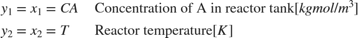The control objective is to maintain the reactor temperatureat its desired setpoint, which changes over time when reactor transitions from low conversion rate to high conversion rate. The coolant temperature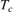is the manipulated variable used by the MPC controller to track the reference as well as reject the measured disturbance arising from the inlet feed stream temperature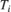. The inlet feed stream concentration,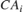, is assumed to be constant. The Simulink model mpc_cstr_plant implements the nonlinear CSTR plant. For more information on the CSTR reactor and related examples, see CSTR Model.

It is well known that the CSTR dynamics are strongly nonlinear with respect to reactor temperature variations and can be open-loop unstable during the transition from one operating condition to another. A single MPC controller designed at a particular operating condition cannot give satisfactory control performance over a wide operating range.

To control the nonlinear CSTR plant with linear MPC control technique, you have a few options:

• If a linear plant model cannot be obtained at run time, first you need to obtain several linear plant models offline at different operating conditions that cover the typical operating range. Next you can choose one of the two approaches to implement MPC control strategy:

(1) Design several MPC controllers offline, one for each plant model. At run time, use Multiple MPC Controller block that switches MPC controllers from one to another based on a desired scheduling strategy. See Gain-Scheduled MPC Control of Nonlinear Chemical Reactor for more details. Use this approach when the plant models have different orders or time delays.

(2) Design one MPC controller offline at the initial operating point. At run time, use Adaptive MPC Controller block (updating predictive model at each control interval) together with Linear Parameter Varying (LPV) System block (supplying linear plant model with a scheduling strategy). See Adaptive MPC Control of Nonlinear Chemical Reactor Using Linear Parameter-Varying System for more details. Use this approach when all the plant models have the same order and time delay.

• If a linear plant model can be obtained at run time, you should use Adaptive MPC Controller block to achieve nonlinear control. There are two typical ways to obtain a linear plant model online:

(1) Use successive linearization. See Adaptive MPC Control of Nonlinear Chemical Reactor Using Successive Linearization for more details. Use this approach when a nonlinear plant model is available and can be linearized at run time.

(2) Use online estimation to identify a linear model when loop is closed, as shown in this example. Use this approach when the plant does not exhibit fast unstable dynamics and when a linear model cannot be obtained from either an LPV system or successive linearization.

### Obtain Linear Plant Model at Initial Operating Condition

To implement an adaptive MPC controller, first you need to design a MPC controller at the initial operating point where CAi is 10 kgmol/m^3, Ti and Tc are 298.15 K.

Create operating point specification.

plant_mdl = 'mpc_cstr_plant'; op = operspec(plant_mdl); 

Feed concentration is known at the initial condition.

op.Inputs(1).u = 10; op.Inputs(1).Known = true; 

Feed temperature is known at the initial condition.

op.Inputs(2).u = 298.15; op.Inputs(2).Known = true; 

Coolant temperature is known at the initial condition.

op.Inputs(3).u = 298.15; op.Inputs(3).Known = true; 

Compute initial condition.

[op_point, op_report] = findop(plant_mdl,op); 
 Operating point search report: --------------------------------- opreport = Operating point search report for the Model mpc_cstr_plant. (Time-Varying Components Evaluated at time t=0) Operating point specifications were successfully met. States: ---------- Min x Max dxMin dx dxMax ___________ ___________ ___________ ___________ ___________ ___________ (1.) mpc_cstr_plant/CSTR/Integrator 0 311.2639 Inf 0 8.1176e-11 0 (2.) mpc_cstr_plant/CSTR/Integrator1 0 8.5698 Inf 0 -6.8709e-12 0 Inputs: ---------- Min u Max ______ ______ ______ (1.) mpc_cstr_plant/CAi 10 10 10 (2.) mpc_cstr_plant/Ti 298.15 298.15 298.15 (3.) mpc_cstr_plant/Tc 298.15 298.15 298.15 Outputs: ---------- Min y Max ________ ________ ________ (1.) mpc_cstr_plant/T -Inf 311.2639 Inf (2.) mpc_cstr_plant/CA -Inf 8.5698 Inf 

Obtain nominal values of x, y and u.

x0 = [op_report.States(1).x;op_report.States(2).x]; y0 = [op_report.Outputs(1).y;op_report.Outputs(2).y]; u0 = [op_report.Inputs(1).u;op_report.Inputs(2).u;op_report.Inputs(3).u]; 

Obtain linear plant model at the initial condition.

sys = linearize(plant_mdl, op_point); 

Drop the first plant input CAi and second output CA because they are not used by MPC.

sys = sys(1,2:3); 

Discretize the plant model because Adaptive MPC controller only accepts a discrete-time plant model.

Ts = 0.5; plant = c2d(sys,Ts); 

### Design MPC Controller

You design an MPC at the initial operating condition. When running in the adaptive mode, the plant model is updated at run time.

Specify signal types used in MPC.

plant.InputGroup.MeasuredDisturbances = 1; plant.InputGroup.ManipulatedVariables = 2; plant.OutputGroup.Measured = 1; plant.InputName = {'Ti','Tc'}; plant.OutputName = {'T'}; 

Create MPC controller with default prediction and control horizons

mpcobj = mpc(plant); 
-->"PredictionHorizon" is empty. Assuming default 10. -->"ControlHorizon" is empty. Assuming default 2. -->"Weights.ManipulatedVariables" is empty. Assuming default 0.00000. -->"Weights.ManipulatedVariablesRate" is empty. Assuming default 0.10000. -->"Weights.OutputVariables" is empty. Assuming default 1.00000. 

Set nominal values in the controller

mpcobj.Model.Nominal = struct('X', x0, 'U', u0(2:3), 'Y', y0(1), 'DX', [0 0]); 

Set scale factors because plant input and output signals have different orders of magnitude

Uscale = [30 50]; Yscale = 50; mpcobj.DV.ScaleFactor = Uscale(1); mpcobj.MV.ScaleFactor = Uscale(2); mpcobj.OV.ScaleFactor = Yscale; 

Due to the physical constraint of coolant jacket, Tc rate of change is bounded by 2 degrees per minute.

mpcobj.MV.RateMin = -2; mpcobj.MV.RateMax = 2; 

Reactor concentration is not directly controlled in this example. If reactor temperature can be successfully controlled, the concentration will achieve desired performance requirement due to the strongly coupling between the two variables.

mdl = 'ampc_cstr_estimation'; open_system(mdl);The model includes three parts:

1. The "CSTR" block implements the nonlinear plant model.

2. The "Adaptive MPC Controller" block runs the designed MPC controller in the adaptive mode.

3. The "Recursive Polynomial Model Estimator" block estimates a two-input (Ti and Tc) and one-output (T) discrete time ARX model based on the measured temperatures. The estimated model is then converted into state space form by the "Model Type Converter" block and fed to the "Adaptive MPC Controller" block at each control interval.

In this example, the initial plant model is used to initialize the online estimator with parameter covariance matrix set to 1. The online estimation method is "Kalman Filter" with noise covariance matrix set to 0.01. The online estimation result is sensitive to these parameters and you can further adjust them to achieve better estimation result.

Both "Recursive Polynomial Model Estimator" and "Model Type Converter" are provided by System Identification Toolbox. You can use the two blocks as a template to develop appropriate online model estimation for your own applications.

The initial value of A(q) and B(q) variables are populated with the numerator and denominator of the initial plant model.

[num, den] = tfdata(plant); Aq = den{1}; Bq = num; 

Note that the new linear plant model must be a discrete time state space system with the same order and sample time as the original plant model has. If the plant has time delay, it must also be same as the original time delay and absorbed into the state space model.

### Validate Adaptive MPC Control Performance

Controller performance is validated against both setpoint tracking and disturbance rejection.

• Tracking: reactor temperature T setpoint transitions from original 311 K (low conversion rate) to 377 K (high conversion rate) kgmol/m^3.

• Regulating: feed temperature Ti has slow fluctuation represented by a sine wave with amplitude of 5 degrees, which is a measured disturbance fed to MPC controller.

Simulate the closed-loop performance.

open_system([mdl '/Concentration']) open_system([mdl '/Temperature']) sim(mdl) 
-->Assuming output disturbance added to measured output #1 is integrated white noise. -->"Model.Noise" is empty. Assuming white noise on each measured output.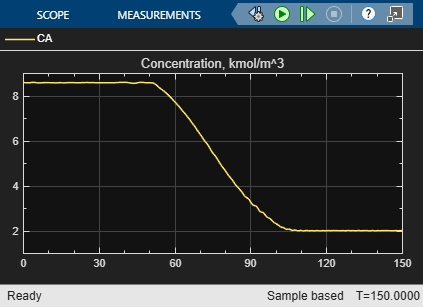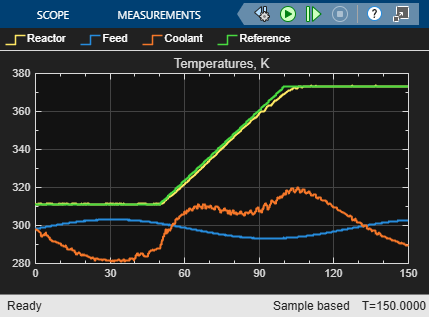The tracking and regulating performance is very satisfactory.

### Compare with Non-Adaptive MPC Control

Adaptive MPC provides superior control performance than non-adaptive MPC. To illustrate this point, the control performance of the same MPC controller running in the non-adaptive mode is shown below. The controller is implemented with a MPC Controller block.

mdl1 = 'ampc_cstr_no_estimation'; open_system(mdl1) open_system([mdl1 '/Concentration']) open_system([mdl1 '/Temperature']) sim(mdl1)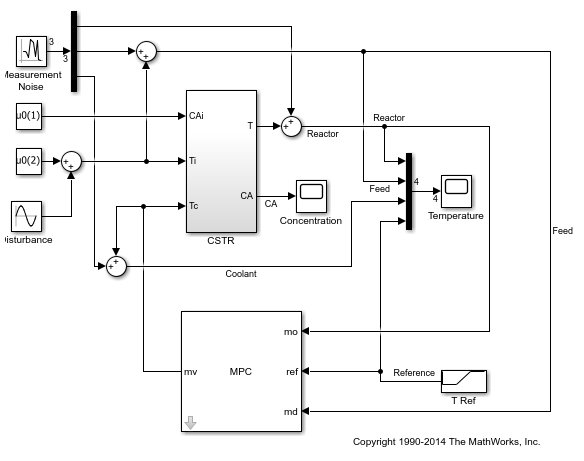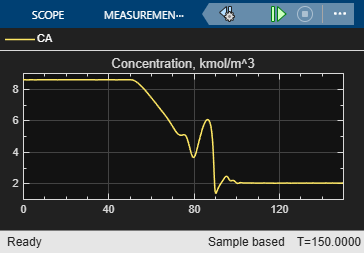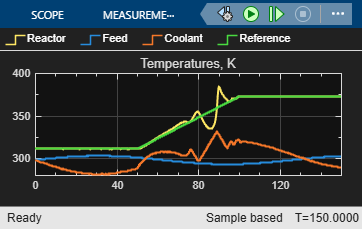As expected, the tracking and regulating performance is unacceptable.

bdclose(mdl) bdclose(mdl1)